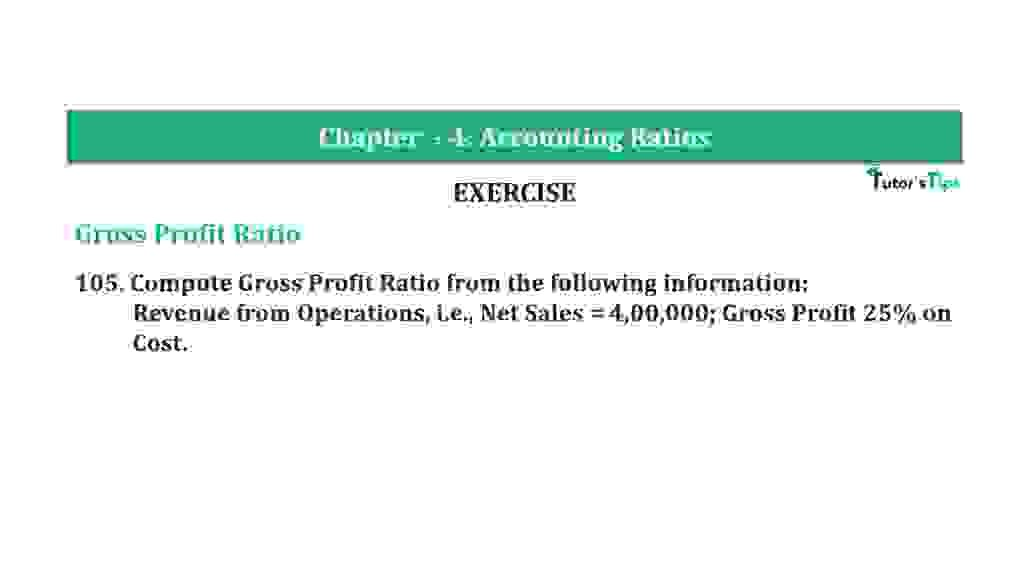# Question 105 Chapter 4 of +2-B – T.S. Grewal 12 ClassQuestion 105 Chapter 4 of +2-B

Gross Profit Ratio

105. Compute Gross Profit Ratio from the following in formation:
Revenue from Operations, i.e., Net Sales = 4,00,000; Gross Profit 25% on
Cost.

### The solution of Question 105 Chapter 4 of +2-B: –

 Gross Profit = 25% on Cost Cost = X

 Gross Profit = X + 25 100 = 25x 100

Sales = Cost + Gross Profit

 Rs. 4,00,000 = X + 25x 100 Rs. 4,00,000 x 100 = 125x Rs. 4,00,00,000 = 125x X = 4,00,00,000 125
 Cost = Rs. 3,20,000 Gross Profit = Sales – Cost = Rs. 4,00,000 – Rs. 3,20,00 = Rs. 80,000
 Revenue from Operations = Gross Profit X 100 Net Sales
 Revenue from Operations = Rs. 80,000 X 100 Rs. 4,00,000 = 20%

Balance Sheet: Meaning, Format & Examples

Thanks, Please Like and share with your friends

Comment if you have any question.

Also, Check out the solved question of previous Chapters: –

### T.S. Grewal’s Double Entry Book Keeping (Vol. II: Accounting for Companies)T.S. Grewal’s Analysis of Financial Statements